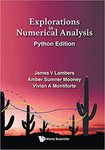## Faculty Books#### Title

Explorations In Numerical Analysis

#### Description

This textbook is intended to introduce advanced undergraduate and early-career graduate students to the field of numerical analysis. This field pertains to the design, analysis, and implementation of algorithms for the approximate solution of mathematical problems that arise in applications spanning science and engineering, and are not practical to solve using analytical techniques such as those taught in courses in calculus, linear algebra or differential equations.

Topics covered include computer arithmetic, error analysis, solution of systems of linear equations, least squares problems, eigenvalue problems, nonlinear equations, optimization, polynomial interpolation and approximation, numerical differentiation and integration, ordinary differential equations, and partial differential equations. For each problem considered, the presentation includes the derivation of solution techniques, analysis of their efficiency, accuracy and robustness, and details of their implementation, illustrated through the Python programming language.

This text is suitable for a year-long sequence in numerical analysis, and can also be used for a one-semester course in numerical linear algebra.

9789811229343

1-18-2021

#### Publisher

WSPCCOinS